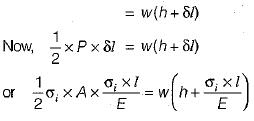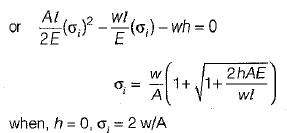Courses

# Test: Design Against Static Load - 1

## 10 Questions MCQ Test Topicwise Question Bank for Mechanical Engineering | Test: Design Against Static Load - 1

Description
This mock test of Test: Design Against Static Load - 1 for Mechanical Engineering helps you for every Mechanical Engineering entrance exam. This contains 10 Multiple Choice Questions for Mechanical Engineering Test: Design Against Static Load - 1 (mcq) to study with solutions a complete question bank. The solved questions answers in this Test: Design Against Static Load - 1 quiz give you a good mix of easy questions and tough questions. Mechanical Engineering students definitely take this Test: Design Against Static Load - 1 exercise for a better result in the exam. You can find other Test: Design Against Static Load - 1 extra questions, long questions & short questions for Mechanical Engineering on EduRev as well by searching above.
QUESTION: 1

### A coil chain of a crane required to carry a maximum load of 100 kN, is shown in following figure.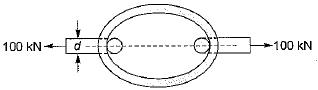What is the diameter of the link stock, if the permissible tensile stress in the link material is not to exceed 150 MPa?

Solution: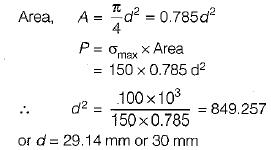QUESTION: 2

### A cast iron link, as shown in following figure, is required to transmit a steady tensile load of 50 kN. What is the maximum tensile stress induced?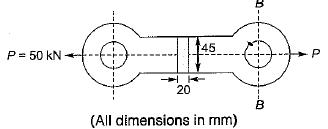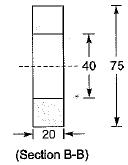Solution:

There are two type of c/s area in the given cast iron link, one is solid c/s (20 mm x 45 mm) and other is hollow c/s at B-B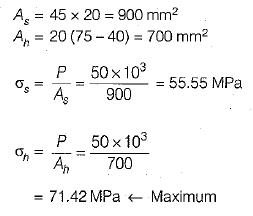QUESTION: 3

### A hydraulic press exerts a total load of 4 MN. This load' is carried by two steel rods, supporting the upper head of the press. If the safe stress is 100 MPa and E =210 kN/mm2, what is the design diameter of supporting steel rods?

Solution: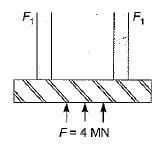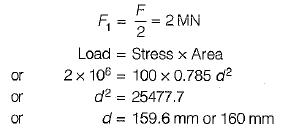QUESTION: 4

What is the force required to punch a circular blank of 50 mm diameter, in a plate of 5 mm thick?

The ultimate shear stress of the plate is 350 N/mm2.

Solution: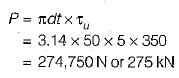QUESTION: 5

A pull of 80 kN is transmitted from a bar X to the bar through a pin as shown in following figure. If the maximum permissible tensile stress in the bars is 100 N/mm2 and the permissible shear stress in the pin is 80 N/mm2, what is the diameter of the pin?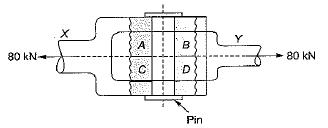Solution: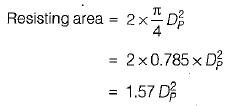Permissible shear stress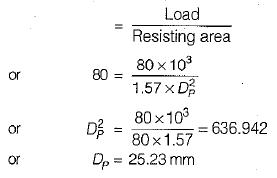QUESTION: 6

A journal 30 mm in diameter supported in sliding bearings has a maximum end reaction of 2500 N. What is the length of sliding bearing for an allowable bearing pressure of 5 N/mm2?

Solution:

Projected area,

A = l x d =30 l mm2

Bearing pressure,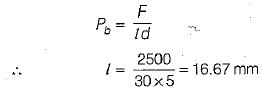QUESTION: 7

A mild steel rod of 15 mm diameter was tested for tensile strength with the gauge length of 60 mm. Following observations were recorded: Final length = 80 mm; Final diameter = 10 mm; Yield load = 4 kN and Ultimate load = 6.5 kN.
Based on the above tensile test data, match the following;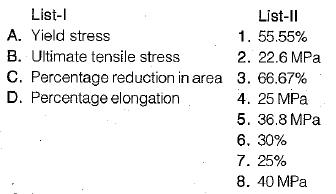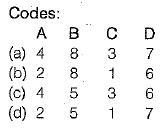Solution:

Original area,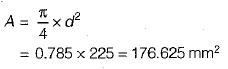Final area,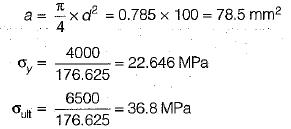% reduction in area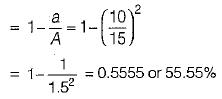% reduction in length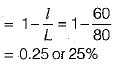QUESTION: 8

Solution:
QUESTION: 9

The ratio of the ultimate stress to the design stress is known as

Solution:
QUESTION: 10

The stress in the bar when load is applied suddenly is Xtimes as compared to the stress induced due to gradually applied load. The value of X is

Solution: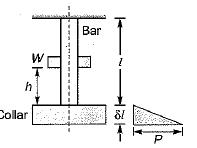The energy gained by the system in the form of strain energy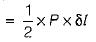The potential energy lost by the weight